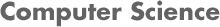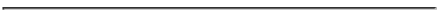### CS539 Machine Learning - Spring 2005  Project 4 - Evaluating Hypotheses

#### PROF. CAROLINA RUIZ

Due Date: Thursday, Feb. 24 2005 at 4 pm.1. Study Chapter 5 in detail.

2. Solve each and every book exercise at the end of the Chapter 5:

5.1, 5.2, 5.3, 5.4, 5.5, and 5.6.

3. Use stratified sampling to select two different subsets of 1000 data instances each from the Cover Type dataset. Piotr's script can be used for this purpose - thanks Piotr! Let's denote these subsets S1 and S2.

1. Learn a J4.8 decision tree t over S1 using a 75% split. That is, use 75% of the data to build the tree and the remaining 25% to calculate the errorS(t). Use this errorS1'(t) to estimate with 95% probability the errorD(t), i.e. the error of t over the entire distribution D of cover type instances.

2. Train a neural network nn with 1 hidden layer and other default parameters over the dataset S2 using a 75% split. That is, use 75% of the data in S2 to build the tree and the remaining 25% to calculate the errorS2'(nn). Compare the decision tree t from above and the neural network nn by estimating the difference d between the true errors of these two hypotheses with 95% probability using errorS1'(t) and errorS2'(nn).

3. Compare J4.8 decision trees and Neural Networks over the Cover Type dataset by estimating the difference in error between J4.8 decision trees and Neural Networks over the Cover Type dataset with an approximate confidence interval of 95%. Do this by using a paired t test with k=11 with the data subset S1 from above as D0.

Please turn in written solutions to these problems at the beginning of class on Thursday, February 24th and be ready to discuss your solutions and the chapter in class.